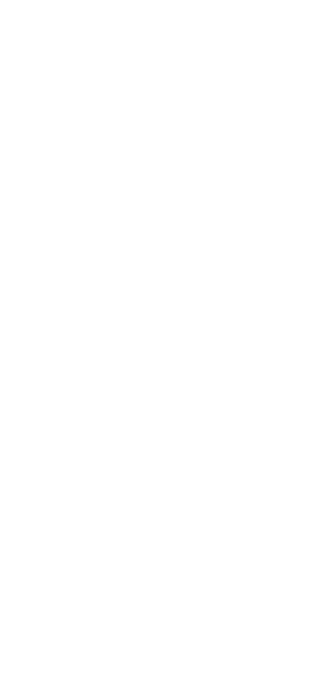# Iteration Notes

## Repeated Elements

Draw four circles by writing four calls to `circle`.

``````function setup() {
createCanvas(windowWidth, windowHeight);
background(100);
}

function draw() {
circle(50, 50, 20);
circle(50, 100, 20);
circle(50, 150, 20);
circle(50, 200, 20);
}``````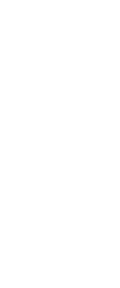``````function draw() {
circle(50, 50, 20);
circle(50, 100, 20);
circle(50, 150, 20);
circle(50, 200, 20);
circle(50, 250, 20);
circle(50, 300, 20);
}``````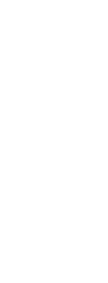There is a simple correspondence between lines of code, and generated graphics.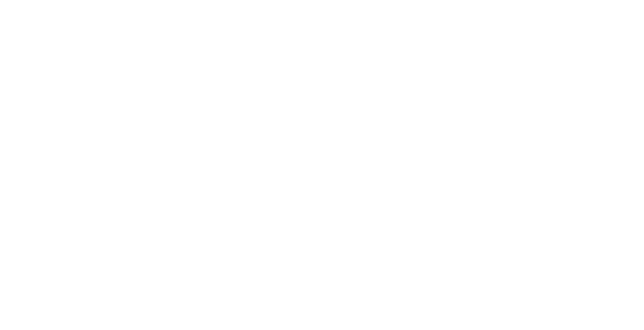It is easy to change just one repeated element.

``````function draw() {
circle(50, 50, 20);
circle(50, 100, 20);
circle(50, 150, 50);
circle(50, 200, 20);
circle(50, 250, 20);
circle(50, 300, 20);
}``````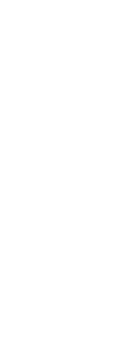Changing many elements requires editing many lines of code.

``````function draw() {
circle(50, 50, 50);
circle(50, 100, 50);
circle(50, 150, 50);
circle(50, 200, 50);
circle(50, 250, 50);
circle(50, 300, 50);
}``````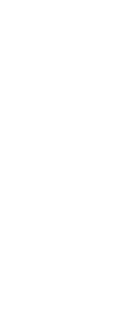Drawing a large number of elements would require a lot of code.

``````function draw() {
circle(50, 50, 50);
circle(50, 100, 50);
circle(50, 150, 50);
circle(50, 200, 50);
circle(50, 250, 50);
circle(50, 300, 50);
// …
}``````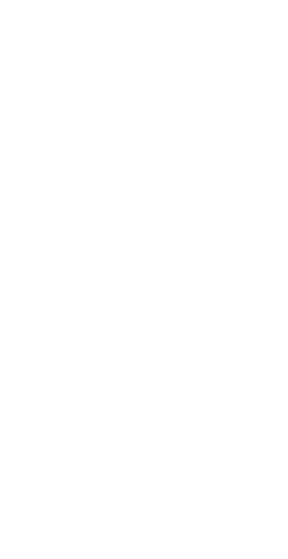# Preparing for iteration

We will prepare the code for iteration the same way that a different sequence prepared it to use functions: by making the repeated sections of source code look more similar to each other.

Let's pare back to four circles, and demonstrate a different way to repeat multiples.

``````function draw() {
circle(50, 50, 40);
circle(50, 100, 40);
circle(50, 150, 40);
circle(50, 200, 40);
}``````Introduce a variable, so that the calls to `circle` all look the same.

``````function draw() {
let y = 50;
circle(50, y, 40);

y = 100;
circle(50, y, 40);

y = 150;
circle(50, y, 40);

y = 200;
circle(50, y, 40);
}``````

Make the lines that set `y` to a new value look the same.

``````function draw() {
let y = 50;
circle(50, y, 40);

y = y + 50;
circle(50, y, 40);

y = y + 50;
circle(50, y, 40);

y = y + 50;
circle(50, y, 40);
}``````

`y += 50` is a shortcut for `y = y + 50`.

``````function draw() {
let y = 50;
circle(50, y, 40);

y += 50;
circle(50, y, 40);

y += 50;
circle(50, y, 40);

y += 50;
circle(50, y, 40);
}``````

Add a final `y += 50`. This doesn't change the behavior because `y` isn't used again. It makes all the stanzas the same, though.

``````function draw() {
let y = 50;

circle(50, y, 40);
y += 50;

circle(50, y, 40);
y += 50;

circle(50, y, 40);
y += 50;

circle(50, y, 40);
y += 50;
}``````

We can use `{` and `}` to group a block of code. This doesn't change its meaning.

``````function draw() {
let y = 50;

{
circle(50, y, 40);
y += 50;
}

{
circle(50, y, 40);
y += 50;
}

{
circle(50, y, 40);
y += 50;
}

{
circle(50, y, 40);
y += 50;
}
}``````

We only want to keep drawing circles until we reach the bottom circle, that has a `y` value of 200. The `if` statements make this explicit. Since `y <= 200` all four times, this code has the same behavior as the previous version. (We would still have to add more code if we wanted the sketch to be able to draw more than four circles.)

``````function draw() {
let y = 50;

if (y <= 200) {
circle(50, y, 40);
y += 50;
}

if (y <= 200) {
circle(50, y, 40);
y += 50;
}

if (y <= 200) {
circle(50, y, 40);
y += 50;
}

if (y <= 200) {
circle(50, y, 40);
y += 50;
}
}``````

`if` means to do a thing zero or one times. `while` means keep doing it: do it zero or more times. Replace the multiple `if` statements by a single `while`.

``````function draw() {
let y = 50;

while (y <= 200) {
circle(50, y, 40);
y += 50;
}
}``````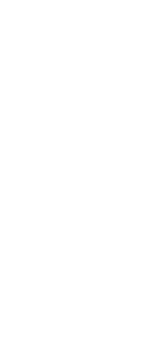Now it's easy to change the spacing ( change `y += 50` to e.g. `y += 80`):

``````function draw() {
let y = 50;

while (y <= 200) {
circle(50, y, 40);
y += 30;
}
}``````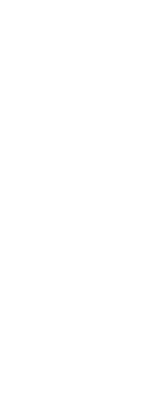Or the termination condition (change `y <= 200` to e.g. `y <= 400`).

``````function draw() {
let y = 50;

while (y <= 400) {
circle(50, y, 40);
y += 80;
}
}``````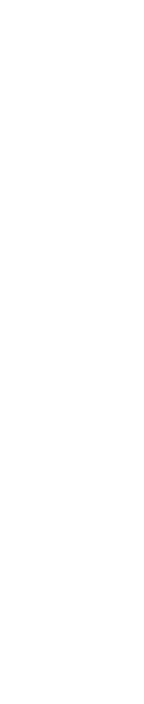These would be bigger changes, if each line of circle were drawn by a different line of code.

# Infinite Loops

It's also easy to write an infinite loop! This `while` statement never finishes, so `draw` never returns.

``````function draw() {
let y = 50;

while (y <= height) {
circle(50, y, 40);
y += 0;
}
}``````

This is also an infinite loop, because when a p5 sketch starts, mouseX and mouseY both have the value 0 the first time that `draw` is called

`mouseX` and `mouseY` are updated between calls to `draw`, so moving the mouse while the loop is running doesn't break out of it.

``````function draw() {
let y = 50;

while (y <= height) {
circle(50, y, 40);
y += mouseY;
}
}``````

A solution. `max(mouseY, 1)` has the value `1` when `mouseY` is less than 1, and the value `mouseY` when `mouseY ≧ 1`.

``````function draw() {
let y = 50;

while (y <= height) {
circle(50, y, 40);
y += max(mouseY, 1);
}
}``````

# The `for` statement

This pattern of using a variable that changes value each time through the loop, and whose value is used in the termination test, is so common that there's a special statement, `for`, that indicates that a loop is used this way.

A `for` statement has the form `for (initialization; test; increment) { body }`. This collects the code that has to do with controlling the loop into a single line.

These two functions are equivalent. They each initial `y` to 50, increment it by 50 each time, draw circles while `y` is less or equal to 200.

``````function draw() {
let y = 50; // initialization

while (y <= 200) { // termination
circle(50, y, 40);
y += 50; // increment
}
}``````
``````function draw() {
for (let y = 50; y <= 200; y += 50) {
circle(50, y, 40);
}
}``````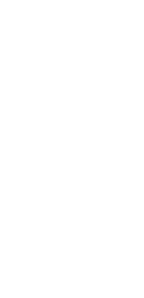We can demonstrate this by writing a single function that uses both forms of loop.

``````function draw() {
for (let y = 50; y <= 800; y += 80) {
circle(50, y, 40);
}

let y = 50;
while (y <= 800) {
circle(150, y, 40);
y += 80;
}
}``````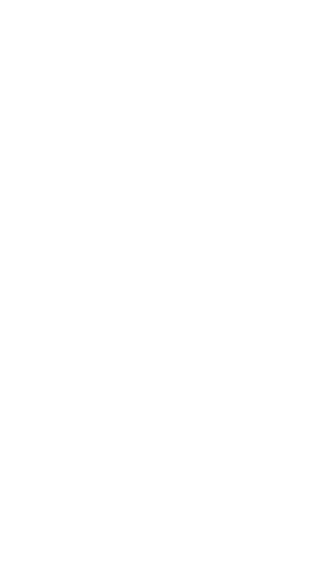# Controlling loop count

The code above repeats circles until they've reached a certain y position.

We can draw an exact number of circles, instead, by using a variable that counts the number of circles that we've drawn.

Let's modify the code from above to count circles. We do this by adding an iteration variable, `i`, that counts the number of circles. The code on the left is the code from above, that draws however many circles it takes to reach the `y` value. The code in the middle and the code on the right shows two different ways to count the number of times through the loop body: by counting from 1 to 10, and by counting from 0 to 9.

Using `while`:

``````function draw() {
let y = 50;

while (y <= 200) {
circle(50, 50, 40);
y += 50;
}
}``````
``````function draw() {
let y = 50;
let i = 1;

while (i <= 10) {
circle(50, 50, 40);
y += 50;
i += 1;
}
}``````
``````function draw() {
let y = 50;
let i = 0;

while (i < 10) {
circle(50, 50, 40);
y += 50;
i += 1;
}
}``````

Using `for`:

``````function draw() {
for (let y = 50; y <= 200; y += 50) {
circle(50, y, 40);
}
}``````
``````function draw() {
let y = 50;
for (let i = 1; i <= 10; i += 1) {
circle(50, y, 40);
y += 50;
}
}``````
``````function draw() {
let y = 50;
for (let i = 0; i < 10; i += 1) {
circle(50, y, 40);
y += 50;
}
}``````

Notes:

• Usually a `for` loop is used for this, rather than `while.`
• Usually `i` is used as the iteration variable. If one loop is inside another one, `i` is used for the outer loop and `j` for the inner one.
• It is common to count from 0 and using `<`, than to count from 1 and use `<=` or `==`. When we cover arrays, we will see why.
• Just as `i += 1` is an abbreviation for `i = i + 1`, `i++` is an abbreviation for `i += 1`. The `for` line in the final sketch above could be written `for (let i = 0; i < 10; i++)`.

# Unrolling the loop

For review: all of the following sketches are equivalent.

``````function draw() {
let y = 50;
for (let i = 0; i < 4; i += 1) {
circle(50, y, 40);
}
}``````
``````function draw() {
let y = 50;
let i = 0;

{
circle(50, y, 40);
y += 50;
i++;
}

{
circle(50, y, 40);
y += 50;
i++;
}

{
circle(50, y, 40);
y += 50;
i++;
}

{
circle(50, y, 40);
y += 50;
i++;
}
}``````
``````function draw() {
let y = 50;
let i = 0;

circle(50, y, 40);
y += 50;
i++;

circle(50, y, 40);
y += 50;
i++;

circle(50, y, 40);
y += 50;
i++;

circle(50, y, 40);
y += 50;
i++;
}``````

Since `i` is not used in the unrolled version, and since we know the value that `y` has at each line, these are also equivalent to:

``````function draw() {
let y = 50;

circle(50, y, 40);
y += 50;

circle(50, y, 40);
y += 50;

circle(50, y, 40);
y += 50;

circle(50, y, 40);
y += 50;
}``````

``````function draw() {
let y = 50;

circle(50, y, 40);
y = 100;

circle(50, y, 40);
y = 150;

circle(50, y, 40);
y = 200;

circle(50, y, 40);
y = 250;
}``````
``````function draw() {
circle(50, 50, 40);
circle(50, 100, 40);
circle(50, 150, 40);
circle(50, 200, 40);
}``````

# Effects

## Counting

This code, similar to the code above increments `y` by a constant amount until it reaches the bottom of the screen:

``````function draw() {
for (let y = 50; y < height; y += 80) {
circle(50, y, 40);
}
}``````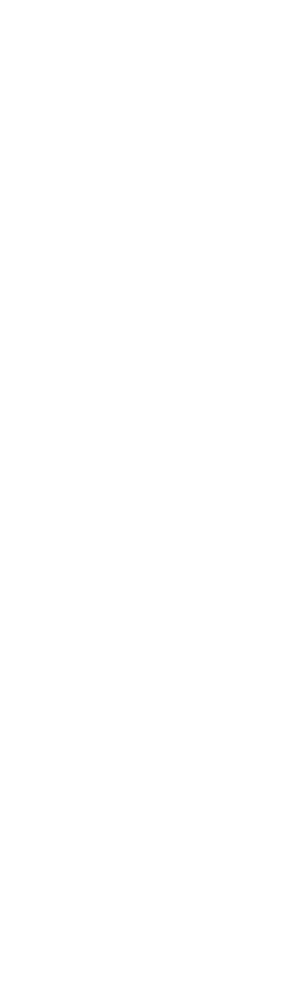We can instead increase `y` by 10% each time through the loop. (`y *= 1.1` is an abbreviation of `y = y * 1.1`, which increases the value of `y` by 10%.)

``````function draw() {
for (let y = 50; y < height; y *= 1.1) {
circle(50, y, 40);
}
}``````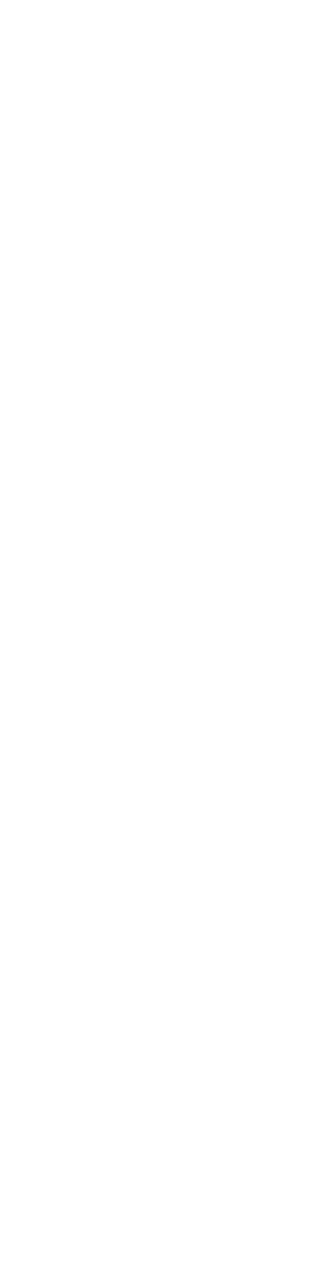## Drawing one shape differently

You may recall that one of the disadvantages of using iteration, instead of one line of code for each element, is that it is more difficult to modify a single element in a repetition.

You can still do this. Here are three different ways to draw one element differently.

``````function draw() {
let y = 50;
for (let i = 0; i < 8; i ++) {
if (i === 3) {
circle(50, y, 60);
} else {
circle(50, y, 40);
}
y += 80;
}
}``````
``````function draw() {
let y = 50;
for (let i = 0; i < 8; i ++) {
let size = 40;
if (i === 3) {
size = 60;
}
circle(50, y, size);
y += 80;
}
}``````
``````function draw() {
let y = 50;
for (let i = 0; i < 8; i ++) {
circle(50, y, i === 3 ? 60 : 40);
y += 80;
}
}``````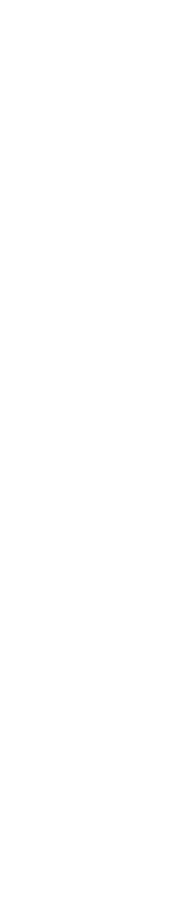## Modifying x and y

We can increase by `x` and `y`.

``````function draw() {
let x = 50;
for (let y = 50; y < height; y *= 1.1) {
circle(x, y, 40);
}
}``````

Since `y` is used to control the loop termination, it makes sense to use it as the iteration variable (above), although we could also do either of these:

``````function draw() {
let x = 50;
let y = 50;
while (y < height) {
circle(x, y, 40);
x += 10;
y *= 1.1
}
}``````
``````function draw() {
for (let y = 50, x = 50; y < height; x += 10, y *= 1.1) {
circle(x, y, 40);
}
}``````